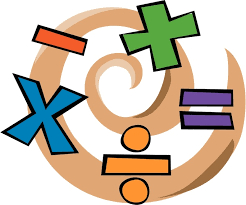# Sum of three fractions

What is the sum of 6/7, 1/2 & 3/4?

x =  59/28 = 2 328

### Step-by-step explanation:Did you find an error or inaccuracy? Feel free to write us. Thank you!

Tips for related online calculators
Need help calculating sum, simplifying, or multiplying fractions? Try our fraction calculator.